## Extreme Value Distribution

N.B. A detailed on-line essay by S. Finch was the starting point for this entry.

Letdenote the extreme'' (i.e., largest) Order Statisticfor a distribution ofelementstaken from a continuous Uniform Distribution. Then the distribution of theis(1)

and the Mean and Variance are(2)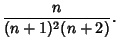(3)

Ifare taken from a Standard Normal Distribution, then its cumulative distribution is(4)

whereis the Normal Distribution Function. The probability distribution ofis then(5)

The Meanand Varianceare expressible in closed form for small,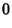(6)(7)(8)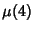(9)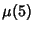(10)

and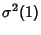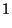(11)(12)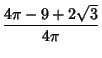(13)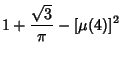(14)(15)

No exact expression is known foror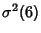, but there is an equation connecting them(16)

An analog to the Central Limit Theorem states that the asymptotic normalized distribution ofsatisfies one of the three distributions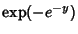(17)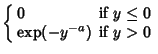(18)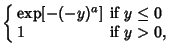(19)

also known as Gumbel, Fréchet, and Weibull Distributions, respectively.

References

Balakrishnan, N. and Cohen, A. C. Order Statistics and Inference. New York: Academic Press, 1991.

David, H. A. Order Statistics, 2nd ed. New York: Wiley, 1981.

Finch, S. Favorite Mathematical Constants.'' http://www.mathsoft.com/asolve/constant/extval/extval.html

Gibbons, J. D. and Chakraborti, S. Nonparametric Statistical Inference, 3rd rev. ext. ed. New York: Dekker, 1992.